2019-08-13 11:15:43 qq_24872841 阅读数 113

## 灰度图定义

1.浮点算法：Gray=R0.3+G0.59+B0.11
2.整数方法：Gray=(R
30+G59+B11)/100
3.移位方法：Gray =(R76+G151+B*28)>>8;
4.平均值法：Gray=（R+G+B）/3;
5.仅取绿色：Gray=G；

``````import numpy as np
import cv2

def gray(image):
h,w = image.shape[:2]             				    #获取图片的high和wide
img = np.zeros([h,w],image.dtype)                   #创建一张和当前图片大小一样的图片
# print(img)
for i in range(h):
for j in range(w):
m = image[i,j]                              #取出当前high和wide中的BGR坐标
img[i,j] = int(m*0.11 + m*0.59 + m*0.3)   #将BGR坐标转化为gray坐标并赋值给新图像
print("image show: %s"%img)
cv2.imshow("image show",img)

dst = cv2.cvtColor(src,cv2.COLOR_BGR2GRAY)             #opencv自带RGB转灰度图api
print("gray: %s"%dst)                                  #查看自带api转灰度图结果的数组
cv2.imshow("gray image",dst)
gray(src)
cv2.waitKey(0)
cv2.destroyAllWindows("image")
``````opencv自带api转换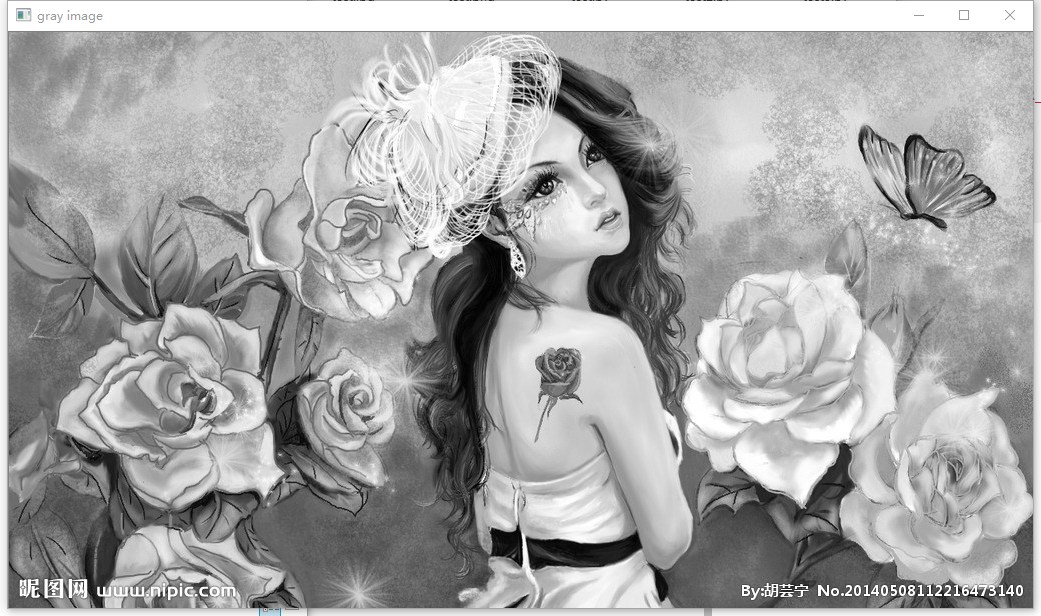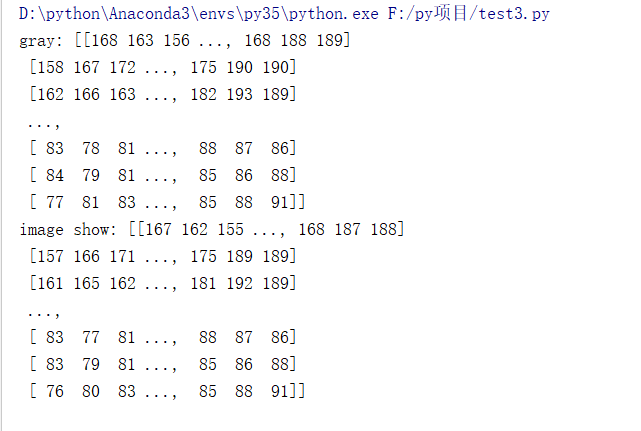2019-10-29 21:00:33 qq_36511401 阅读数 6307

1、介绍。

通道。三通道一般指彩色图片，分别为R、G、B通道，我们所见的彩色图片，则是这三个通道叠加的效果。四通道一般是指在三通道的基础上再加上一个透明度的通道。单通道一般指灰度图，但是三通道如果每个像素的三个通道值都相等的话，我们也称为灰度图。

灰度图。Gray Scale Image 或是Grey Scale Image，又称灰阶图。把白色与黑色之间按对数关系分为若干等级，称为灰度。灰度分为256阶。因为彩色图片每个像素有三个字节（24bit）的值，怎么样才能取到一字节（8bit）的灰度等级呢。我们一般有7种方法：最小值法、最大值法、平均值法、加权法、红色值法、绿色值法和蓝色值法。

下面我会介绍这7种方法，以下图所示的源图为例。2、代码。生成两个文件夹，一个存放单通道的图像，另一个存放是三通道的图像。每个文件夹都会各生成7种不同方法灰度化处理的图像。至于使用哪种方法，要看具体的例子。

``````import javax.imageio.ImageIO;
import java.awt.image.BufferedImage;
import java.io.File;

public class ImgTest {
private static final byte Gray_Type_Min = 1;//最大值法
private static final byte Gray_Type_Max = 2;//最小值法
private static final byte Gray_Type_Average = 3;//平均值法
private static final byte Gray_Type_Weight = 4;//加权法
private static final byte Gray_Type_Red = 5;//红色值法
private static final byte Gray_Type_Green = 6;//绿色值法
private static final byte Gray_Type_Blue = 7;//蓝色值法

private static final String File_Path = "G:\\xiaojie-java-test\\img\\%s\\%s.jpg";
private static final String Source_Path = "G:\\xiaojie-java-test\\img\\source.jpg";

public static void main(String[] args) {
//图片灰度化
toGrayImg(Gray_Type_Min, "gray_min");//最大值法
toGrayImg(Gray_Type_Max, "gray_max");//最小值法
toGrayImg(Gray_Type_Average, "gray_average");//平均值法
toGrayImg(Gray_Type_Weight, "gray_weight");//加权法
toGrayImg(Gray_Type_Red, "gray_color_red");//红色值法
toGrayImg(Gray_Type_Green, "gray_color_green");//绿色值法
toGrayImg(Gray_Type_Blue, "gray_color_blue");//蓝色值法
}

/**
* 图片灰度化的方法
*
* @param type         灰度化方法
* @param grayFileName 目标图片文件名
*/
private static void toGrayImg(int type, String grayFileName) {
try {
final int imgWidth = image.getWidth();
final int imgHeight = image.getHeight();
BufferedImage bufferedImage_1 = new BufferedImage(imgWidth, imgHeight, BufferedImage.TYPE_BYTE_GRAY);
BufferedImage bufferedImage_3 = new BufferedImage(imgWidth, imgHeight, BufferedImage.TYPE_INT_RGB);
//这边因为只是灰度操作，所以内外循环imgWidth和imgHeight可以随便放
for (int i = 0; i < imgHeight; i++) {
for (int j = 0; j < imgWidth; j++) {
final int pixel = image.getRGB(j, i);
final int[] grb = getRgb(pixel);
final int gray = getGray(grb, type);
bufferedImage_1.setRGB(j, i, (byte) gray);//这里一定要用byte类型的，不然图片会很暗
bufferedImage_3.setRGB(j, i, getPixel(gray, gray, gray));
//System.out.print(String.format("%4d ", gray));
}
//System.out.println();
}
ImageIO.write(bufferedImage_1, "JPEG", new File(String.format(File_Path, "单通道-灰度图", grayFileName)));
ImageIO.write(bufferedImage_3, "JPEG", new File(String.format(File_Path, "三通道-灰度图", grayFileName)));
} catch (Exception e) {
e.printStackTrace();
}
}

//将一个int值转化为3个r、g个b的byte值
private static int[] getRgb(int pixel) {
int[] rgb = new int;
rgb = (pixel >> 16) & 0xff;
rgb = (pixel >> 8) & 0xff;
rgb = pixel & 0xff;
return rgb;
}

//将3个r、g个b的byte值转化为一个int值
private static int getPixel(int r, int g, int b) {
int getPixel = b;
getPixel += (r << 16);
getPixel += (g << 8);
return getPixel;
}

//根据不同的灰度化方法，返回byte灰度值
private static int getGray(int[] rgb, int type) {
if (type == Gray_Type_Average) {
return (rgb + rgb + rgb) / 3;   //rgb之和除以3
} else if (type == Gray_Type_Weight) {
return (int) (0.3 * rgb + 0.59 * rgb + 0.11 * rgb);
} else if (type == Gray_Type_Red) {
return rgb;//取红色值
} else if (type == Gray_Type_Green) {
return rgb;//取绿色值
} else if (type == Gray_Type_Blue) {
return rgb;//取蓝色值
}
//比较三个数的大小
int gray = rgb;
for (int i = 1; i < rgb.length; i++) {
if (type == Gray_Type_Min) {
if (gray > rgb[i]) {
gray = rgb[i];//取最小值
}
} else if (type == Gray_Type_Max) {
if (gray < rgb[i]) {
gray = rgb[i];//取最大值
}
}
}
return gray;
}
}
``````

3、结果。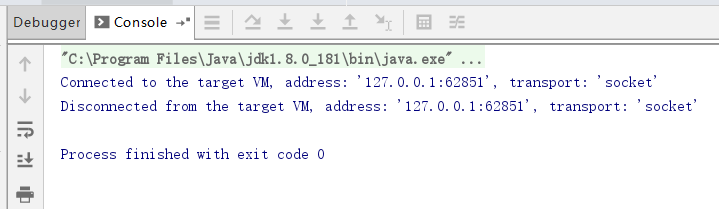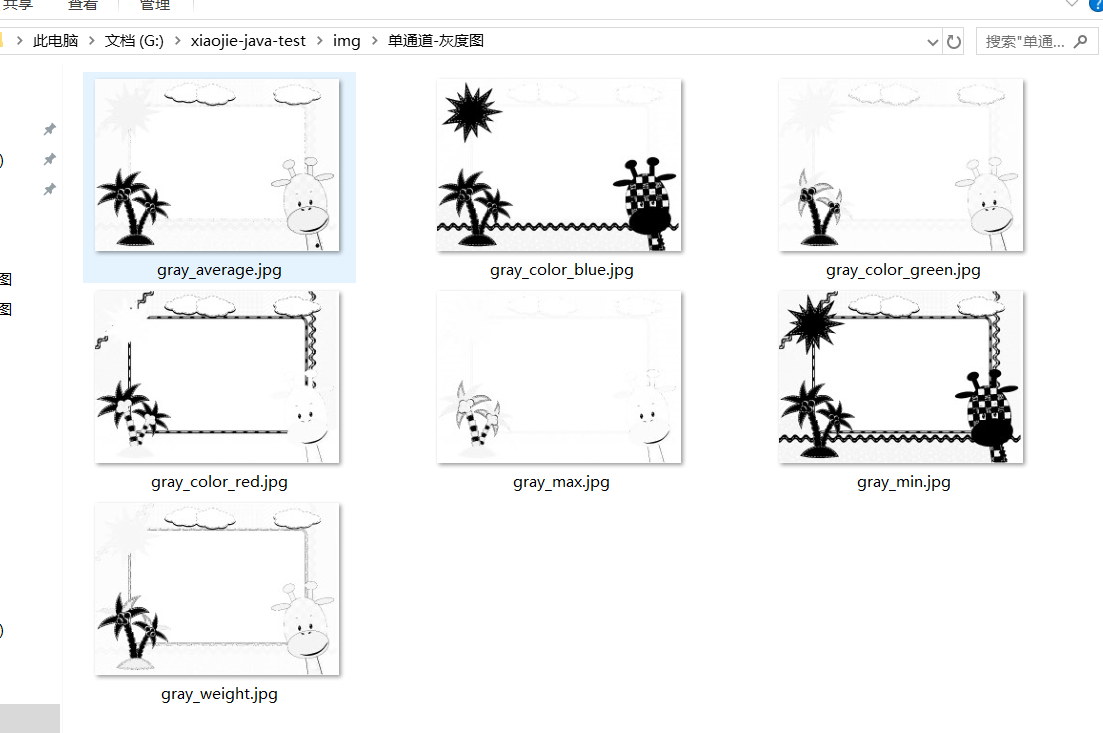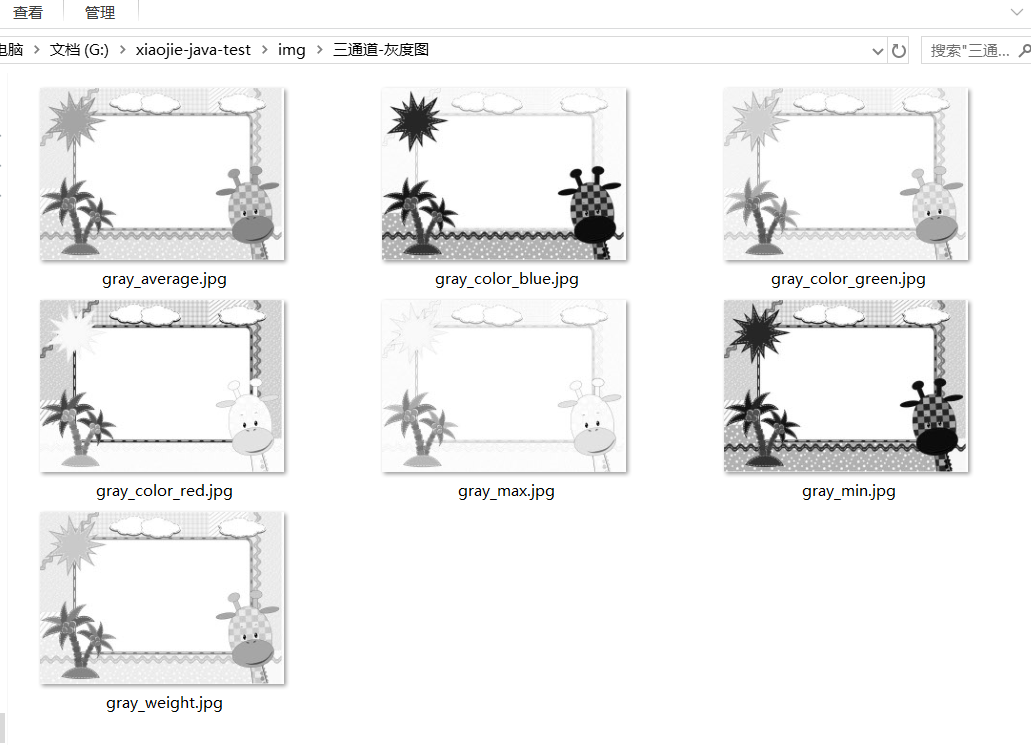2012-09-23 02:15:33 sm_crystal 阅读数 2944

## 进阶

2015-12-16 16:53:33 shenziheng1 阅读数 1671

1.灰阶图像形态学

2.灰阶图像形态学基本运算

2.1 Grayscale Dilation(膨胀)

2.1.1 数学表达F=(7 9 8 3 8 9 9); K=(-3 0* -3)  【其实质就是空间位移变动与灰度变动的过程】 *：代表中心点

-3 ：7 9 8 3 8 9 9                 左移一位

4 6 5 0 5 6 6 # #           灰度变化

0* ：# 7 9 8 3 8 9 9 #            中心位置，不用平移，直接灰度改变

-3 ：# # 4 6 5 0 5 6 6            右移一位，叠加灰度值2.2 Erosion(腐蚀)F=(7 9 8 3 8 9 9); K=(-3 0* -3)  【其实质就是空间位移变动与灰度变动的过程】 *：代表中心点

-3 ：#   #  7     9    8   3    8   9   9                 右移一位

#   #  10  12  11  6   11 12 12            灰度变化

0* ：#   7   9    8   3    8   9   9    #            中心位置，不用平移，直接灰度改变

-3 ：10 12 11  6  11  12  12  #   #           左移一位，叠加灰度值2.3 Opening(开运算)2.4 Closing(闭运算)2.5 四种基本运算进行总结对比1.四种运算都会对图像的纹理，进行一个平滑。Dilation和Closing对于细节进行了明显膨胀，图像整体灰度增加；Erosion和Opening对“细节”进行了明显的腐蚀，在图像灰度波动频繁的区域操作，会变暗，变得平滑。

2.由于一幅图像大部分区域都是平缓的。我们可以看出，Erosion和Dilation操作在平滑图像的基础上，对图像的整体都进行了腐蚀或者膨胀。而Closing和Opening运算却不一样，仅仅对灰度变化极其频繁（频率大）的区域进行平滑，对图像的缓和区（大部分区域）没有任何影响。

3.基本运算的Matlab仿真以及结果分析

3.1  Dilation实验3.2 Erosion 实验3.3 Opening实验3.4 Closing实验2013-04-08 21:34:36 mlkiller 阅读数 1457

# 一 灰度图定义

Gray Scale Image 或是Grey Scale Image，又称灰阶图。把白色黑色之间按对数关系分为若干等级，称为灰度。灰 度分为256阶。用灰度表示的图像称作灰度图

1.浮点算法：Gray=R*0.3+G*0.59+B*0.11
2.整数方法：Gray=(R*30+G*59+B*11)/100
3.移位方法：Gray =(R*76+G*151+B*28)>>8;
4.平均值法：Gray=（R+G+B）/3;
5.仅取绿色：Gray=G；

# 二 灰度的计算

`out.create(input.cols,input.rows,CV_8UC1);`

``` int i,j;
uchar* p;
uchar* q;
uchar R,G,B;
for( i = 0; i < nRows; ++i)
{
p = input.ptr<uchar>(i);
q = out.ptr<uchar>(i);
for ( j = 0; j < nCols; ++j)
{
B = p[j * nChannels];
G =  p[j * nChannels + 1];
R =  p[j * nChannels + 2];
q[j] = 0.299 * R + 0.587 * G + 0.114*B;

}
}```

公式二：Gray =(R*76+G*151+B*28)>>8;

只是把上面的那句话替换掉。

# 三 效果和效率系统方法 乘法 移位